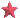Teaching Interests
 Calculus Differential Equations Operations Research Variational Analysis Probability Real Analysis Wavelets Financial Mathematics Linear Algebra Difference Equations Statistics Control Theory Numerical Analysis Complex Analysis Stock Pricing Theory Environmental EconomicsResearchTeachingLinksHome